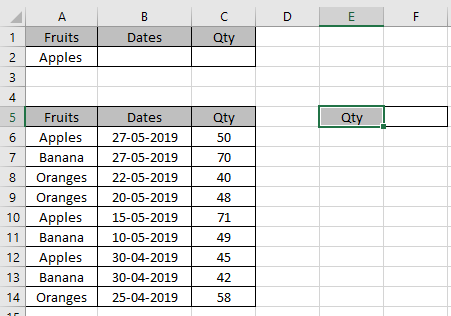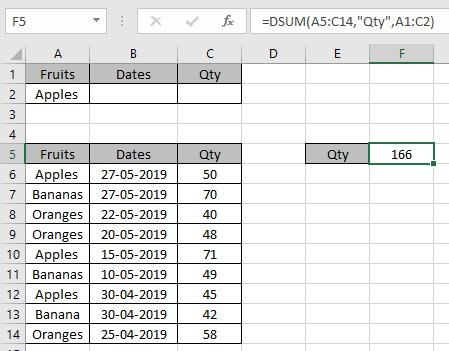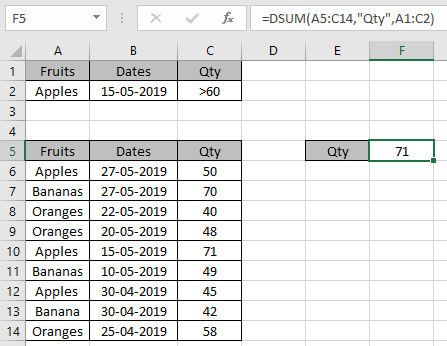# How to use the DSUM function in excel

DSUM function is built-in SUM function in Excel. DSUM function returns the SUM of the field based on given many criteria. The DSUM function takes criteria as a table.
Syntax:

=DSUM (database, field, criteria)

Database : table as an array
Field : the required field, call by name or text in quotes.
Criteria : one or more conditions taken as table array.

This all might be confusing to you so lets gear up and understand this function using it in an example.Here we have a list of fruits bought with their respective weights and I need to get a few SUM of Qty based on criterias.

There are some things you have to take care of when using this function

1. Database and criteria table should have same fields.
2. Formula must be used in any other cell than criteria area.

First condition is total quantity of Apples bought
Use the formula:

=DSUM (A5:C14, "Qty", A1:C2)

A5:C14 : database table as an array
"Qty" : field by text in quotes or field can be input as 3 (3rd column in the table).
A1:C2 : criteria as table arrayThe total sum of Apples is 166.

DSUM function can have multiple criterias in one formula.

Now we will take an example taking multiple criterias.You don’t have to use the formula because criteria field gets updated as soon as you give input in criteria.

Wildcards keys ( *, ? , ~) can be used with the DSUM function.

It will get you the SUM of the values.

As you can see the DSUM function in excel returns values from the table field.

Hope you understood How to use the DSUM function in Excel. Explore more articles on Mathematical functions like SUMIF and SUMIFS functions here. Please state your query or feedback in the comment box below.

Related Articles

How to use the DCOUNT function in Excel

How to use the DSUM function in Excel

How to use Wildcards in Excel

Popular Articles:

How to use the VLOOKUP Function in Excel

How to use the COUNTIF in Excel 2016

How to use the SUMIF Function in Excel

Edit a dropdown list

If with conditional formatting

Vlookup by date

Terms and Conditions of use

The applications/code on this site are distributed as is and without warranties or liability. In no event shall the owner of the copyrights, or the authors of the applications/code be liable for any loss of profit, any problems or any damage resulting from the use or evaluation of the applications/code.